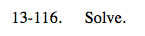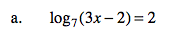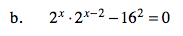### Home > A2C > Chapter 13 > Lesson 13.2.2 > Problem13-116

13-116.
1. Solve. Homework Help ✎

1. log7(3x − 2) = 2

2. 2x − 2x−2 − 162 = 03x − 2 = 7216 = 24

x = 5